## A point in many triangles

#### Abstract

We give a simpler proof of the result of Boros and Füredi that for any finite set of points in the plane in general position there is a point lying in 2/9 of all the triangles determined by these points.

### Introduction

Every set P of n points in Rd in general position determines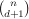d-simplices. Let p be another point in Rd. Let C(P,p) be the number of the simplices containing p. Boros and Füredi  constructed a set P of n points in R2 for which C(P,p)≤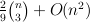for every point p. They also proved that there is always a point p for which C(P,p)≥for every point p. Here we present a new simpler proof of the existence of such a point p.

### Proof

Let P be a set of n points in the plane. By the extension of a theorem of Buck and Buck  due to Ceder  there are three concurrent lines that divide the plane into 6 parts each containing at least n/6-1 points in its interior. Denote by p the point of intersection of the three lines. Every choice of six points, one from each of the six parts, determines a hexagon containing the point p.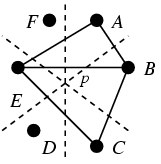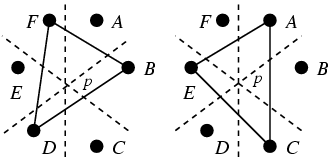Figure 1: a)p∈ABE or p∈BCE b)p∈ACE and p∈BDF

Among the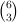triangles determined by the vertices of the hexagon, at least 8 triangles contain the point p. Indeed, from each of the six pairs of triangles situated as in Figure 1a we get one triangle containing p. In addition, p is contained in both triangles of the Figure 1b. Therefore, by double counting, the number of triangles containing p is at least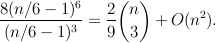For the sake of completeness we include a sketch of a proof of the modification of the theorem of Buck and Buck that we used above.

Proposition 1. Let μ be a finite measure absolutely continuous with respect to the Lebesgue measure on R2. Then there are three concurrent lines that partition the plane into six parts of equal measure.

The partition theorem for the finite set of point P follows by letting μ be the restriction of the Lebesgue measure to the union of tiny disks of equal size centered at the points of P. Since P is in general position, none of the three lines passes through more than two of the disks.

Proof sketch. The given measure can be made into one which gives every open set a strictly positive measure, and which differs little from the given one. Proving the result for the latter, and using a compactness argument, one is through. Hence we can assume the property mentioned, and we normalize the total measure of the plane to 1.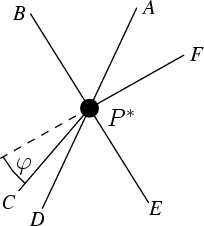Figure 2: Six rays

Let now u be a unit vector. There is a unique directed line L(u) pointing in the direction u and cutting the plane in two parts of measure 1/2. For any point P on L(u) there are six unique rays from P, denoted A(u,P),…,F(u,P) in clockwise order, splitting the plane in sectors of measure 1/6, with A(u,P) in the direction u. Note that L(u) is the union of A(u,P) and D(u,P). When P moves along L(u) in the direction u, the ray B(u,P) will turn counterclockwise in a continuous way, becoming orthogonal to L(u) at some point. As the clockwise turning E(u,P) behaves in the same way, there will be a unique P*(u) such that B(u,P*(u)) and E(u,P*(u)) form a line.

The line L, the point P* and the six rays from P* clearly depend continuously on u. In particular the angle φ(u) one must turn C(u,P*(u)) counterclockwise to complete F(u,P*) to a line varies continuously. But for any u, we have C(-u,P*(-u))=F(u,P*(u)), and hence φ(-u)=-φ(u). This shows that for some v the angle φ(v) vanishes and the rays C(v,P*(v)) and F(v,P*(v)) form a line. This finishes the proof. □

For no dimension higher than 2 the optimal bounds for C(P,p) are known. Bárány  showed that there is always a point p for which C(P,p)≥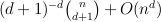.

Acknowledgement. I thank the referee for comments that resulted in much improved proof of proposition 1.

### References

1
I. Bárány, A generalization of Carathéodory's theorem, Discrete Math. 40 (1982), 141–152.
2
E. Boros and Z. Füredi, The number of triangles covering the center of an n-set, Geom. Dedicate 17 (1984), 69–77.
3
R. C. Buck and E. F. Buck, Equipartition of convex sets, Math. Mag 22 (1949), 195–198.
4
J. G. Ceder, Generalized sixpartite problems, Bol. Soc. Mat. Mexicana (2) 9 (1964), 28–32.